# Texas Go Math Grade 2 Lesson 17.4 Answer Key Partition Rectangles

Refer to our Texas Go Math Grade 2 Answer Key Pdf to score good marks in the exams. Test yourself by practicing the problems from Texas Go Math Grade 2 Lesson 17.4 Answer Key Partition Rectangles.

## Texas Go Math Grade 2 Lesson 17.4 Answer Key Partition Rectangles

Essential Question
Have you find the total number of same-size squares that will cover a rectangle?
Explanation:
Number of rows x number of columns = area

Explore
Put several color tiles together. Trace around the shape to draw a two-dimensional shape.
Explanation: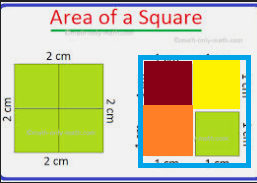Kept several squares to make a full square.
Drawn a two dimensional shape.

Math Talk
Mathematical Processes
Is there a different shape that can be made with the same number of tiles? Explain.
Explanation:
Yes, We can make a polygon with the help of these shapes.Home Connection

• After puffing together tiles, your child traced around them to draw a two-dimensional shape, This activity is an introduction to partitioning a rectangle into several same-size squares.Explanation:
The above rectangle is divided into equal number of squares
2 x 6 = 12
Number of rows x number of columns = area

Model and Draw
Trace around color tiles. How many square units cover this rectangle?

Each color tile is 1 square unit.Number of rows: 2
Number of columns: 3
Total: _____ square unitsExplanation:
6 square units
Number of rows x number of columns = area
6 square units cover this rectangle

Share and Show

Use color tiles to cover the rectangle.
Trace around the square tiles. Write how many.

Question 1.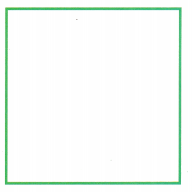Number of rows: _____
Number of columns: ____
Total: ___ square units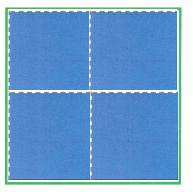Explanation:
Number of rows: 2
Number of columns: 2
Total: 4 square units

Question 2.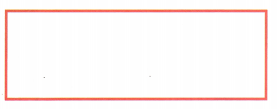Number of rows: ___
Number of columns: ____
Total: ___ square unitsExplanation:
Number of rows: 1
Number of columns: 3
Total: 3 square units

Problem Solving

Use color tiles to cover the rectangle. Trace around the square tiles. Write how many.

Question 3.Number of rows: ___
Number of columns: ___
Total: __ square units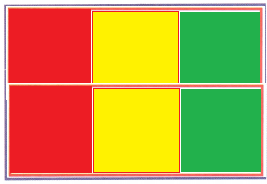Explanation:
Number of rows: 2
Number of columns: 3
Total: 6 square units

Question 4.
H.O.T. Multi-Step Mary started to cover this rectangle with ones blocks. Explain how you would estimate the number of ones blocks that would cover the whole rectangle.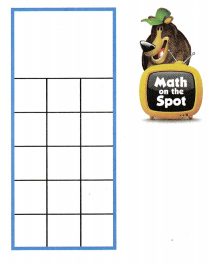Explanation:
Number of rows: 7
Number of columns: 3
Total: 21 square units
number of ones blocks that would cover the whole rectangle is 21

Question 5.
H.O.T. Reggie drew the shape at the right. How many color tiles would cover the shape?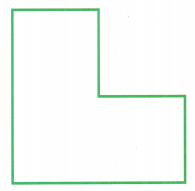__ color tiles.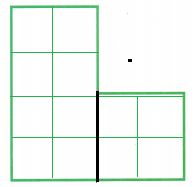Explanation:
2 x 4 = 8
2 x 2 = 4
8 + 4 = 12 color tiles.

Question 6.
Use color tiles to cover the rectangle. Trace around the square tiles.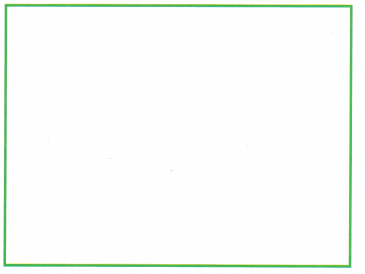Which of the following is the size of the rectangle?
(A) 7 square units
(B) 12 square units
(C) 10 square units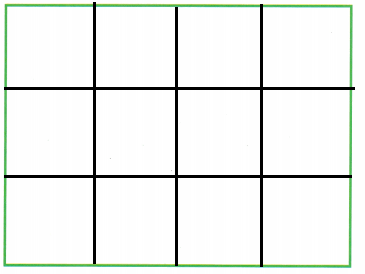Explanation:
3 x 4 = 12
12 square units is the size of the rectangle

Question 7.
Texas Test Prep A rectangle is covered with color tiles. There are 4 rows of 2 color tiles. How many color tiles cover the shape?
4 x 2 = 8
Explanation:
A rectangle is covered with color tiles.
There are 4 rows of 2 color tiles.
8 color tiles cover the shape.

Take Home Activity

• Have your child describe what he or she did in this lesson.
Explanation:
Covered the two dimensional shapes
with colored squares

### Texas Go Math Grade 2 Lesson 17.4 Homework and Practice Answer Key

Color the square tiles. Write how many.

Question 1.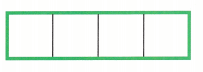Number of rows: ____
Number of columns: ____
Total: _____ square units
Number of rows: 1
Number of columns: 4
Total: 4 square units
Explanation:
Area = number of rows x number of columns
1 x 4 = 4 square units

Question 2.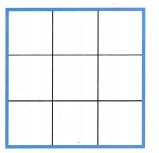Number of rows: ____
Number of columns: ____
Total: _____ square units
Explanation:
Area = number of rows x number of columns
1 x 4 = 4 square units

Question 3.
Multi-Step Brenda started to cover this rectangle with square tiles. Explain how you would estimate the number of square tiles that would cover the whole rectangle.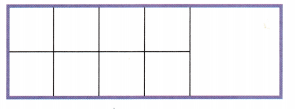Explanation:
Area = number of rows x number of columns
2 x 6 = 12 square tiles
Brenda started to cover this rectangle with square tiles.
the number of square tiles that would cover the whole rectangle is 12.

Texas test Prep

Question 4.
A rectangle is covered with color tiles. There are 5 rows and 2 columns. How many color tiles cover the shape?
(A) 12
(B) 10
(C) 8
Explanation:
Area = number of rows x number of columns
5 x  2 = 10

Question 5.
Sal uses color tiles to make a rectangle. He makes 3 rows and 6 columns. How many color tiles does Sal use?
(A) 16
(B) 15
(C) 18
Explanation:
Area = number of rows x number of columns
3 x 6 = 18
Sal uses color tiles to make a rectangle.
He makes 3 rows and 6 columns.

Question 6.
Emily covers a rectangle with square tiles. She makes 4 columns and 4 rows. What is the size of the rectangle?
(A) 16 square units
(B) 12 square units
(C) 20 square units
Explanation:
Emily covers a rectangle with square tiles.
She makes 4 columns and 4 rows
4 x 4 = 16
Area = number of rows x number of columns
The size of the rectangle is 16 square units

Question 7.
Multi-Step Frank has 9 color tiles. He wants to make a rectangle with 5 rows and 3 columns of color tiles. How many more color tiles does Frank need?
(A) 6
(B) 3
(C) 7